Refer to our Texas Go Math Grade 8 Answer Key Pdf to score good marks in the exams. Test yourself by practicing the problems from Texas Go Math Grade 8 Unit 3 Study Guide Review Answer Key.

Module 7 Angle Relationships in Parallel Lines and Triangles

Question 1.
If m∠GHA = 76°, find the measures of the given angles. (Lesson 7.1)m∠EGC = _____________
m∠EGD = _____________
m∠BHF = _____________
m∠HGD = _____________
(i) ∠GHA = ∠EGC (corresponding angles) = 106°
(ii) ∠EGC + ∠EGD = 180°
∠EGD = 180 – 106 = 74°
(iii) ∠GHA = ∠BHF (vertical angles) = 106°
(iv) ∠GHA = ∠HGD (alternate interior angles) = 106°

Question 2.
Find the measure of the missing angles. (Lesson 7.2)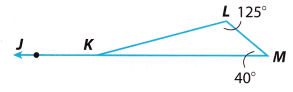m∠JKL = _____________
m∠LKM = _____________
From the Triangle Sum Theorem we have:
m∠L + m∠M + m∠LKM = 1800
We substitute the given angle measures and we solve for x.
125° + 40° + m∠LKM = 180°
165° + m∠LKM = 180°
165° – 165° + m∠LKM = 180° – 165°
m∠LKM = 15°
∠JKL and ∠LKM are supplementary angles, therefore
m∠JKL + m∠LKM = 180°
m∠JKL + 150 = 180°
m∠JKL + 15° – 15° = 180° 15°
m∠JKL = 165°

Question 3.
Is the larger triangle similar to the smaller triangle? Explain your answer. (Lesson 7.3)
yes; the triangles are similar because all of their angles are congruent both triangles have a right angle and angle B.

Question 4.
Find the value of x and y in the figure. (Lesson 7.3)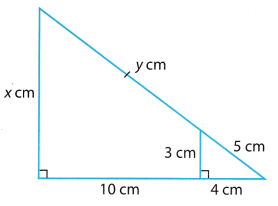In similar triangles, corresponding side lengths are proportional.Also,Module 8 The Pythagorean Theorem

Find the missing side lengths. Round your answers to the nearest hundredth. (Lesson 8.1)

Question 1.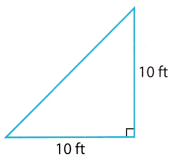Base = 10 ft
Height 10 ft
Hypotenuse = $$\sqrt{\text { base }^{2}+\text { height }^{2}}$$
Hyp = $$\sqrt{10^{2}+10^{2}}$$
Hyp = $$\sqrt{100+100}$$
Hyp = $$\sqrt{200}$$
Hyp = $$10 \sqrt{2}$$ ft

Question 2.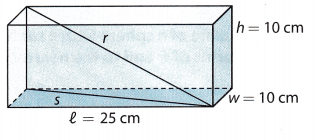We want to find r, the length from a bottom corner to the opposite top corner. First, we find s, the length of the diagonal across the bottom of the box.
w2 + l2 = s2
102 + 252 = s2
100 + 625 = s2
725 = s2
s ≈ 26.9 cm
We use our expression for s to find r.
h2 + s2 = r2
102 + 725 = r2
100 + 725 = r2
825 = r2
r ≈ 28.7 cm

Question 3.
Hye Sun has a modern coffee table whose top is a triangle with the following side lengths: 8 feet, 6 feet, and 5 feet. Is Hye Sun’s coffee table top a right triangle? (Lesson 8.2)
Formula for the right-angle triangle = a² + b² = c²
a = 6 feet
b = 5 feet
c = 8 feet
= 6² + 5² = 8²
= 36 + 25 = 64
= 61 > 64
The given triangle is not a right-angled triangle.

Question 4.
Find the length of each side of triangle ABC. If necessary, round your answers to the nearest hundredth. (Lesson 8.3)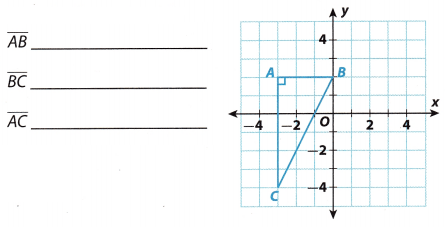The Length of the vertical. leg is 6 units.
$$\overline{A C}$$ = 6 units
The length of the horizontaL leg is 3 units
$$\overline{A B}$$ = 3 units
Let a = 6 and b = 3. Let C represent the Length of the hypotenuse. We use the Pythagorean Theorem to find C.
a2 + b2 = c2
62 + 32 = c2
36 + 9 = c2
45 = c2
c = $$\sqrt {45}$$
c ≈ 6.71
$$\overline{B C}$$ = 6.71 units

Module 9 Volume

Find the volume of each figure. Round your answers to the nearest hundredth. (Lessons 9.1, 9.2, 9.3)

Question 1.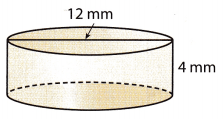Base diameter = 12 mm
Base radius r = $$\frac{12}{2}$$
Base radius r = 6 mm
Height = 4 mm
VoLume of a cylinder = πr2h
V = 3.14 × 62 × 4
V = 3.14 × 36 × 6
V = 452.16 mm3

Question 2.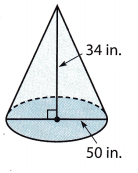Base diameter 50 in
Base radius r = $$\frac{50}{2}$$
Base radius r = 25 in
Height = 34 in
VoIime of a cone = $$\frac{1}{3}$$πr2h
V = $$\frac{1}{3}$$ × 3.14 × 252 × 34
V = 22241.66667 in3
V ≈ 22241.67 in3

Question 3.
Find the volume of a ball with a radius of 1.68 inches.
The ball is in the shape of a sphere
The volume of the sphere = V = 4/3πr².sphere
= 4/3 × π × (1.68) ²
= 4/3 × 3.14 × 2.82
= 5.025 sq. inches.
The volume of the sphere = V = 5.025 sq. inches.

Module 10 Surface Area

Find the lateral and total surface area of each figure. If necessary, round your answers to the nearest hundredth. (Lessons 10.1, 10.2)

Question 1.Given that the shape is the cylinder.
The formula for the total surface area of a cylinder = 2πrh + 2πr².
Diameter = 5ft =5/2
Height = 5ft
= 2(3.14) (2.5) (5) + 2(3.14) (5) ²
= 78.5 + 157
= 235.5 sq. ft.
The total surface area of a cylinder = 235.5 sq. ft.
The lateral surface area of a cylinder = 2πrh
= 2(3.14)(2.5)(5)
= 78.5 sq. ft
The lateral surface area of a cylinder = 78.5 sq. ft.

Question 2.Given that the shape is the rectangular prism
The formula for the surface area of a rectangular prism = 2(lb + bh + lh).
Length = l = 7.5ft
Height = h = 2ft
= 2(7.5 × 4 + 4 × 2 + 7.5 × 2).
= 2(30 + 8 + 15)
= 2(53)
= 106 cu. ft
The surface area of a rectangular prism = 106 cubic ft.
The lateral surface area of the rectangular prism = 2(l + b)h.
= 2(7.5 + 4) × 2.
= 60 × 2
= 120 sq. ft
The lateral surface area of the rectangular prism = 120 sq. ft

Question 3.
A cylindrical water tower has a height of 50 meters and a radius of 20 meters.
Given that,
The height of the cylindrical water tank = 50 meters.
The radius of the cylindrical water tank = 20 meters.
The formula for the total surface area of a cylinder = 2πrh + 2πr².
= 2 × 3.14 × 20 × 50 + 2 × 3.14 × (20)².
= 6280 + 2512
= 8792 sq. meter.
The total surface area of a cylinder = 8792 sq. meter
The lateral surface area of a cylinder = 2πrh
= 2(3.14)(20)(50)
= 6280 sq. meter
The lateral surface area of a cylinder = 6280 sq. meters.

Question 1.
CAREERS IN MATH Hydrologist A hydrologist needs to estimate the mass of water in an underground aquifer, which is roughly cylindrical in shape. The diameter of the aquifer is 65 meters, and its depth is 8 meters. One cubic meter of water has a mass of about 1000 kilograms.

a. The aquifer is completely filled with water. What is the total mass of the water in the aquifer? Explain how you found your answer. Use 3.14 for π and round your answer to the nearest kilogram.
a) Since the aquifer is completely filled with water, we need to find the volume of the aquifer Its diameter is 65 meters, which means that its radius is 32-5 meters.
V = πr2h
V ≈ 3.14 ∙ 32.52 ∙ 8
V ≈ 26, 533m3
Since one cubic meter of water has a mass of about 1000 kiLograms, then we have
26. 533 ∙ 1000 = 26, 533,000kg
The aquifer has about 26,533,000 kg of water

b. Another cylindrical aquifer has a diameter of 70 meters and a depth of 9 meters. The mass of the water in it is 2.7 × 107 kilograms. Is the aquifer totally filled with water? Explain your reasoning.
The diameter of the other aquifer is 70 meters, which means that its radius is 35 meters.
V = πr2h
V ≈ 3.14 ∙ 352 ∙ 9
V ≈ 34,618.5m3
Since one cubic meter of water has a mass of about 1000 kiLograms, then we have
34,618.5 ∙ 1000 = 34, 618, 500 kg
On the other hand, we are told that there are 27 ∙ 107 kg of water in the aquifer, which is more than the aquifer can hold.

Question 2.
From his home, Myles walked his dog north 5 blocks, east 2 blocks, and then stopped at a drinking fountain. He then walked north 3 more blocks and east 4 more blocks, It started to rain so he cut through a field and walked straight home.
a. Draw a diagram of his path.b. How many blocks did Myles walk in all? How much longer was his walk before it started to rain than his walk home?
Before it started to rain, Myles walked 14 bLocks (black route).
5 + 2 + 3 + 4 = 14
To find how many bLocks did he waLk after it started to rain, we let a = 8 blocks and b = 6 blocks. Let C represent the Length of the hypotenuse (the red route, the road straight home). We use the Pythagorean Theorem to find C.
a2 + b2 = c2
82 + 62 = c2
64 + 36 = c2
100 = c2
c = $$\sqrt {100}$$
c = 10 blocks
Myles walked 24 bLocks in total (14 from home to the destination and 10 back home). The walk before it started to rain was 4 blocks longer than the walk home.

Texas Go Math Grade 8 Unit 3 Mixed Review Texas Test Prep Answer Key

Selected Response

Question 1.
Which of the following angle pairs formed by a transversal that intersects two parallel lines are not congruent?
(A) alternate interior angles
(C) corresponding angles
(D) alternate exterior angles

Question 2.
The measures of the three angles of a triangle are given by 3x + 1, 2x – 3, and 9x. What is the measure of the smallest angle?
(A) 13°
(B) 23°
(C) 29°
(D) 40°
(B) 23°

Explanation:
The angles of the given triangle = {(3x + 1), (2x-3), 9x}
As we know that the sum of all the three angles of a triangle is 180°
Thus the sum of the given angles should be also 180°
Therefore,
(3x + 1) + (2x – 3)9x = 180°
3x + 2x + 9x + 1 – 3 = 180
14x – 2 = 180
14x = 182
x = $$\frac{182}{14}$$
x = 13°.
Thus the angles are;
3x + 1 = 3(13) + 1 = 40°
2x – 3 = 2(13) – 3 = 23°
9x = 9(13) = 117°
Since 23° < 40° < 117°
Therefore the smallest angle is 23°.

Question 3.
Using 3.14 for π, what is the volume of the cylinder?(A) 200 cubic yards
(B) 628 cubic yards
(C) 1256 cubic yards
(D) 2512 cubic yards
(B) 628 cubic yards

Explanation:
Diameter of base 10 yd
Radius = $$\frac{10}{2}$$
Height = 8yd
Volume of cylinder = πr2h
Volume = 3.14 × (5)2 × 8
Volume 628 yd3

Question 4.
Which of the following is not true?
(A) $$\sqrt {36}$$ + 2 > $$\sqrt {16}$$ + 5
(B) 5π > 17
(C) $$\sqrt {10}$$ + 1 < $$\frac{9}{2}$$
(D) 5 – $$\sqrt {35}$$ < 0 Answer: (A) $$\sqrt {36}$$ + 2 > $$\sqrt {16}$$ + 5

Explanation:
(A) $$\sqrt {36}$$ + 2 > $$\sqrt {16}$$ + 5
6 + 2 > 4 + 5
8 > 9
This statement is false as 8 is not greater than 9.

(B) 5π > 17
5 ∙ 3.14 < 17
15.7 < 17
This statement is true as 17 is greater than 15.7.

(C) $$\sqrt {10}$$ + 1 < $$\frac{9}{2}$$
3.16 + 1 < 4.5
4.16 < 4.5
This statement is true as 4.5 is greater than 4.16.

(D) 5 – $$\sqrt {35}$$ < 0
5 – 5.92 < 0
5 – 5.92 < 0
-0.92 < 0
This statement is true as 0 is greater than -0.92.

Question 5.
A pole is 65 feet tall. A support wire is attached to the top of the pole and secured to the ground 33 feet from the pole’s base. Find the approximate length of the wire.
(A) 32 feet
(B) 73 feet
(C) 56 feet
(D) 60 feet
(B) 73 feet

Explanation:
Let a = 65 feet and b = 33 feet Let C represent the length of the hypotenuse, the length of the wire. We use the Pythagorean Theorem to find C.
a2 + b2 = c2
652 + 332 = c2
4225 + 1089 = c2
5314 = c2
c = $$\sqrt {5314}$$
c ≈ 72.897
c ≈ 73 feet

Question 6.
Using 3.14 for π, what is the volume of the sphere 7 cm to the nearest tenth?(A) 205.1 cm3
(B) 1077 cm3
(C) 179.5 cm3
(D) 4308.1 cm3
(C) 179.5 cm3

Explanation:
Diameter of base = 7 cm
Radius = $$\frac{7}{2}$$ cm
Volume of sphere = $$\frac{4}{3}$$πr3
Volume = $$\frac{4}{3}$$ × 3.14 × (3.5)3
Volume = 179.5033 cm3
Volume ≈ 179.5cm3

Question 7.
Which set of lengths are not the side lengths of a right triangle?
(A) 28, 45, 53
(B) 13, 84, 85
(C) 36, 77, 85
(D) 16, 61, 65
(D) 16, 61, 65

Explanation:
Check if the lengths in Option A form a right triangle.
Let a = 28, b = 45 and c = 53. Using the converse of the Pythagorean Theorem, we have:
a2 + b2 = c2
282 + 452 = 532
784 + 2025 = 2809
2809 = 2809
True
Since 282 + 452 = 532, the triangle is a right triangle.

Check if the lengths in Option B form a right triangle.
Let a. = 13, b = 84 and c = 85. Using the converse of the Pythagorean Theorem, we have:
a2 + b2 = c2
132 + 842 = 852
169 + 7056 = 7225
7225 = 7225
True
Since 132 + 842 = 852, the triangle is a right triangle.

Check if the Lengths in Option C form a right triangLe.
Let a = 36, b = 77 and c = 85. Using the converse of the Pythagorean Theorem, we have:
a2 + b2 = c2
362 + 772 = 852
1296 + 5929 = 7225
7225 = 7225
True
Since 362 + 772 = 852, the triangle is a right triangle.

Check if the Lengths in Option D form a right triangle.
Let a = 16, b = 61 and c = 65. Using the converse of the Pythagorean Theorem, we have:
a2 + b2 = c2
162 + 612 = 652
256 + 3721 = 4225
3977 = 4225
False
Since 162 + 612 = 652, the triangle is not a right triangle.

Question 8.
Which statement describes the solution of a system of linear equations for two lines with different slopes and different y-intercepts?
(A) one non-zero solution
(B) infinitely many solutions
(C) no solution
(D) solution of 0
(A) one non-zero solution

Question 9.
What is the side length of a cube that has a surface area of 486 square inches?
(A) 7 inches
(B) 8 inches
(C) 9 inches
(D) 10 inches
(C) 9 inches

Explanation:
Volume of the given cube 729 in2
Volume of a cube of side 7 in 73 = 343
Volume of a cube of side 8 in 83 = 512
Volume of a cube of side 9 in 93 = 729
Volume of a cube of side 10 in = 103 = 1000
A cube of side 9 in will have volume of 729 in3. Thus option C is correct.

Question 10.
A box in the shape of a triangular prism is shown below. What is the surface area of the box?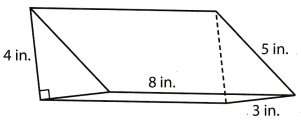(A) 96 in2
(B) 102 in2
(C) 108 in2
(D) 116 in2
Given that the box is in the shape of a triangular prism.
The formula of the surface area of the triangular prism = bh + (b1 + b2 + b3) l.
b = 3 inches.
h = 4 inches.
b1 = 4 inches.
b2 = 3 inches.
b3 = 5 inches.
l = 8 inches.
= 3 × 4 + (4 + 3 + 5)8
= 108 sq. in.
The surface area of the triangular prism = 108 sq. in.
Option B is the correct answer

Gridded Response

Question 11.
What is the value of h in the triangle below?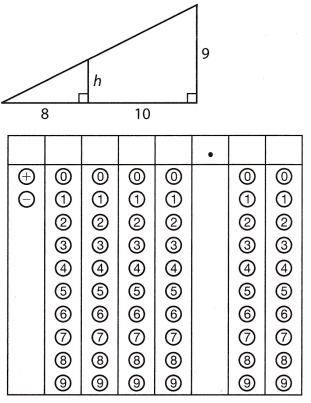In similar triangles the corresponding sides and lengths are proportional.
h/8 = 9/10
h/8 = 0.9
h = 0.9 × 8
h = 7.2
The value of h in the triangle is 7.2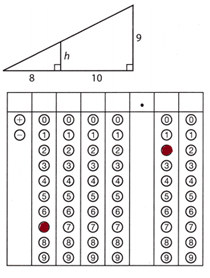Question 12.
Chandra is working on an art project using a cone that has a height of 11 inches and a base radius of 2 inches. Using 3.14 for π, what is the volume of the cone to the nearest tenth of a cubic inch?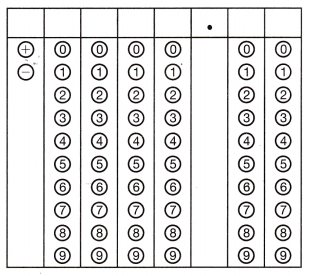Given that the shape is a cone.
The formula for the volume of the cone = 1/3hπr².
Height = h = 11 inches.
Radius = r = 2 inches.
= ⅓ × 11 × 3.14 × (2) ².
= 45.59 sq. inches.
The volume of the cone = 45.59 sq. inches.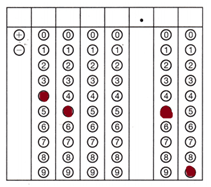Hot Tips! Read graphs and diagrams carefully. Look at the labels for important information.

Question 13.
The net of a cylinder is shown below.Using 3.14 for π, what is the lateral surface area of the cylinder in square meters?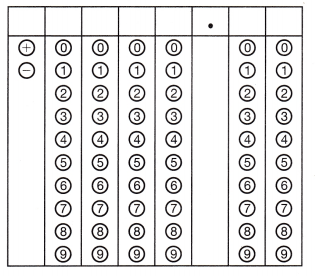Use the puzzle to preview key vocabulary from this unit. Unscramble the circled letters to answer the riddle at the bottom of the page.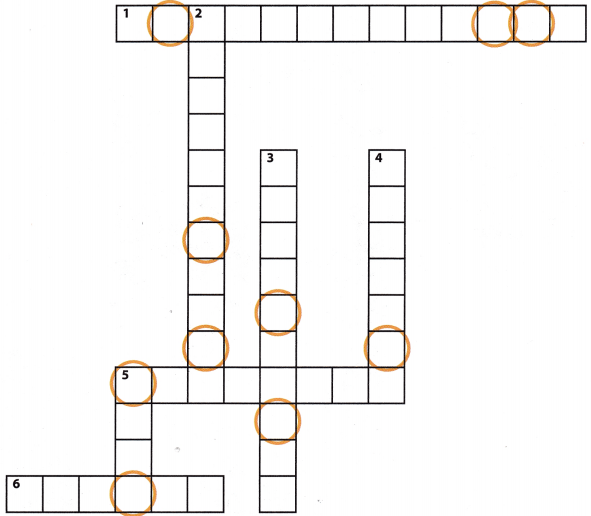Across
1. The angle formed by two sides of a triangle (2 words) (Lesson 7-2)
5. A three-dimensional figure that has two congruent circular bases. (Lesson 9-1)
6. A three-dimensional figure with all points the same distance from the center. (Lesson 9-3)

Down
2. The line that intersects two or more lines. (Lesson 7-1)
3. The side opposite the right angle ¡n a right triangle. (Lesson 8-1)
4. Figures with the same shape but not necessarily the same size. (Lesson 7-3)
5. A three-dimensional figure that has one vertex and one circular base. (Lesson 9-2)

Question.
What do you call an angle that is adorable?